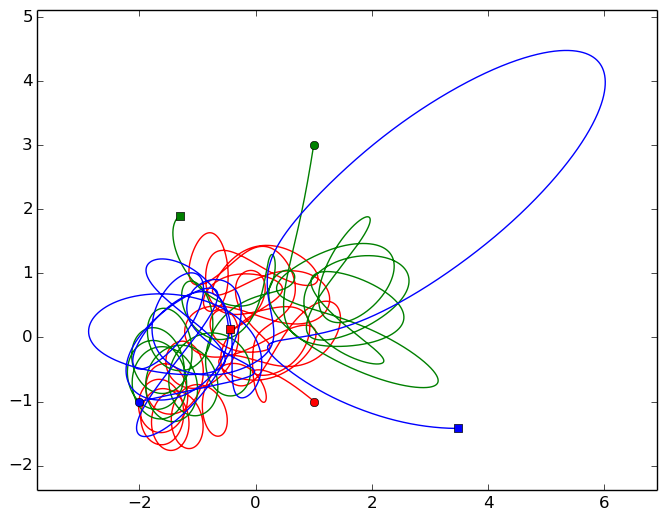# 3 Body Problem in Julia¶

This is a test of the animation capabilities of Julia. I'm going to simulate the 3-body problem in 2D. This is largely based on

http://nbviewer.ipython.org/github/pjpmarques/Julia-Modeling-the-World/blob/master/Three-Body%20Problem.ipynb

but I've done the animation in Julia.

We start by calling the relevant packages.

In :
using PyPlot
using ODE
using PyCall
@pyimport matplotlib.animation as anim

INFO: Loading help data...


The system of ODEs we are gong to solve will be of the form:

$$\frac{d^2\mathbf{r}_i}{dt^2} = \sum_j G\frac{m_j}{||\mathbf{r}_i-\mathbf{r}_j||^3}(\mathbf{r}_i-\mathbf{r}_j)$$

where of course each of the $\mathbf{r}_i$ are position vectors for each planet.

We'll turn this into a first order system by considering the equivalent set of equations:

$$\frac{d\mathbf{r}_i}{dt} = \mathbf{v}_i$$

with $$\frac{d\mathbf{v}_i}{dt} = \sum_j G\frac{m_j}{||\mathbf{r}_i-\mathbf{r}_j||^3}(\mathbf{r}_i-\mathbf{r}_j)$$

We'll encode all of the position and velocity vectors in one vector $y$, in the format:

$$y = [\text{First Planet's Position}, \text{First Planet's Velocity}, \text{Second Planet's Position}, \text{Second Planet's Velocity},\ldots]$$

To start, we must define the right hand side of the sytem above, as this is what the ODE solver requires.

In :
function F(t,y)

#Extract current position and velocity
r1, v1 = y[1:2], y[3:4]
r2, v2 = y[5:6], y[7:8]
r3, v3 = y[9:10], y[11:12]

#Give spatial derivatives
dr1dt = v1
dr2dt = v2
dr3dt = v3

#Work out velocity derivatives (ie accelerations)
dv1dt = -(G*m2*(r1-r2)/(norm(r1-r2)^3) + G*m3*(r1-r3)/(norm(r1-r3)^3))
dv2dt = -(G*m1*(r2-r1)/(norm(r2-r1)^3) + G*m3*(r2-r3)/(norm(r2-r3)^3))
dv3dt = -(G*m1*(r3-r1)/(norm(r3-r1)^3) + G*m2*(r3-r2)/(norm(r3-r2)^3))

#Combine back together into dydt vector
dydt = [dr1dt,dv1dt,dr2dt,dv2dt,dr3dt,dv3dt]

return dydt
end

Out:
F (generic function with 1 method)

We can now set the simulation parameters and integrate the equations of motion.

In :
#Set the planetary masses
m1 = 5
m2 = 4
m3 = 3

#Set the gravitational field strength
G = 1

#Set initial positions and velocities
r10 = [1.01,-1];    v10 =  [0.0,0.0]
r20 = [1.0,3.0];    v20 =  [0.0,0.0]
r30 = [-2.0,-1.0];  v30 =  [0.0,0.0]

#Specify simulation time and steps per second ('steps per second' is only for animation purposes, as ode23s is an adaptive solver.)
tf = 50
stepsPerUnitTime = 500

#Solve the system
y0 = [r10,v10,r20,v20,r30,v30]
tspan = linspace(0,tf,tf*stepsPerUnitTime)
t,y = ode23s(F, y0, tspan; points=:specified);

#Extract the data into a useful form
ymat= hcat(y...)
r1x = ymat[1,:]
r1y = ymat[2,:]
r2x = ymat[5,:]
r2y = ymat[6,:]
r3x = ymat[9,:]
r3y = ymat[10,:]
r1x = reshape(r1x, length(r1x))
r1y = reshape(r1y, length(r1y))
r2x = reshape(r2x, length(r2x))
r2y = reshape(r2y, length(r2y))
r3x = reshape(r3x, length(r3x))
r3y = reshape(r3y, length(r3y));


## Plot¶

Plotting in Julia using PyPlot is quite straightforward, especially if you're a Matplotlib user.

In :
#Find Axis Limits
xmin = minimum([r1x,r2x,r3x])
xmax = maximum([r1x,r2x,r3x])
xmin, xmax = xmin - 0.1(xmax-xmin), xmax+ 0.1*(xmax-xmin)
ymin = minimum([r1y,r2y,r3y])
ymax = maximum([r1y,r2y,r3y])
ymin, ymax = ymin - 0.1(ymax-ymin), ymax+ 0.1*(ymax-ymin)

#Construct Figure and Plot Data
fig = figure()
ax = plt.axes(xlim = (xmin,xmax),ylim=(ymin,ymax))
ax[:plot](r1x,r1y, "r-")
ax[:plot](r2x,r2y, "g-")
ax[:plot](r3x,r3y, "b-")

#Plot start and end points
ax[:plot](r1x,r1y, "ro")
ax[:plot](r1x[end],r1y[end], "rs")
ax[:plot](r2x,r2y, "go")
ax[:plot](r2x[end],r2y[end], "gs")
ax[:plot](r3x,r3y, "bo")
ax[:plot](r3x[end],r3y[end], "bs")Out:
1-element Array{Any,1}:
PyObject <matplotlib.lines.Line2D object at 0x7f81a5a27d50>

## Animation¶

PyPlot's animation syntax is very similar to matplotlib, but there are some idiosyncrasies:

1. We write ax[:plot] rather than ax.plot
2. The animate function takes an input i, which increases by $1$ each frame. However, on the first frame $i = 0$, rather than $1$, because this is Python, not Julia. The simplest way to avoid confusion is to define $k = i+1$ as I have done.
3. Rather than writing

line, = ax.plot([], [])

we write

line = ax[:plot]([],[]).

I find Matplotlib's animation tools very intuitive and powerful, and it's fantastic that they're so easy to use in Julia. I haven't explained all the details of the animation below, because it's so similar to standard matplotlib. A great tutorial on making animations with Matplotlib is here:

https://jakevdp.github.io/blog/2012/08/18/matplotlib-animation-tutorial/

In :
#Use External Viewer for Animation
pygui(true)

#Find Axis Limits
xmin = minimum([r1x,r2x,r3x])
xmax = maximum([r1x,r2x,r3x])
xmin, xmax = xmin - 0.1(xmax-xmin), xmax+ 0.1*(xmax-xmin)
ymin = minimum([r1y,r2y,r3y])
ymax = maximum([r1y,r2y,r3y])
ymin, ymax = ymin - 0.1(ymax-ymin), ymax+ 0.1*(ymax-ymin)

#Construct Figure and Plot Data
fig = figure()
ax = plt.axes(xlim = (xmin,xmax),ylim=(ymin,ymax))
global line1 = ax[:plot]([],[],"r-")
global line2 = ax[:plot]([],[],"g-")
global line3 = ax[:plot]([],[],"b-")
global p1 = ax[:plot]([],[],"or")
global p2 = ax[:plot]([],[],"og")
global p3 = ax[:plot]([],[],"ob")

function init()
global line1
global line2
global line3
global p1
global p2
global p3
line1[:set_data]([],[])
line2[:set_data]([],[])
line3[:set_data]([],[])
p1[:set_data]([],[])
p2[:set_data]([],[])
p3[:set_data]([],[])
return (line1,line2,line3,p1,p2,p3,None)
end

step = 15
function animate(i)
k = i + 1
global line1
global line2
global line3
global p1
global p2
global p3
line1[:set_data](r1x[max(1,step*(k-50)):(step*k)],r1y[max(1,step*(k-50)):(step*k)])
line2[:set_data](r2x[max(1,step*(k-50)):(step*k)],r2y[max(1,step*(k-50)):(step*k)])
line3[:set_data](r3x[max(1,step*(k-50)):(step*k)],r3y[max(1,step*(k-50)):(step*k)])
p1[:set_data]([r1x[step*k]],r1y[step*k])
p2[:set_data]([r2x[step*k]],r2y[step*k])
p3[:set_data]([r3x[step*k]],r3y[step*k])
return (line1,line2,line3,None)
end

#Call the animator.
myanim = anim.FuncAnimation(fig, animate, init_func=init, frames=ifloor(length(t)/step), interval=20)

#This will require ffmpeg or equivalent.
#myanim[:save]("3Body2D.mp4", bitrate=-1, extra_args=["-vcodec", "libx264", "-pix_fmt", "yuv420p"])

Out:
PyObject <matplotlib.animation.FuncAnimation object at 0x7f8182ea06d0>

## Example Animation¶

In :
# Function for creating an embedded video given a filename
function html_video(filename)